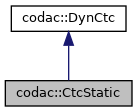codac
codac::CtcStatic Class Reference

Generic static $\mathcal{C}$ that contracts a tube $[\mathbf{x}](\cdot)$ with some IBEX contractor (for boxes, possibly including time). The contractor will be applied on each slice and gate. More...

#include <codac_CtcStatic.h>

Inheritance diagram for codac::CtcStatic:[legend]
Collaboration diagram for codac::CtcStatic:[legend]

## Public Member Functions

CtcStatic (Ctc &ibex_ctc, bool temporal_ctc=false)
Creates a static contractor object $\mathcal{C}$ for tubes. More...

void contract (TubeVector &x)
$\mathcal{C}\big([\mathbf{x}](\cdot)\big)$ More...

void contract (Tube &x1)
$\mathcal{C}\big([x_1](\cdot)\big)$ More...

void contract (Tube &x1, Tube &x2)
$\mathcal{C}\big([x_1](\cdot),[x_2](\cdot)\big)$ More...

void contract (Tube &x1, Tube &x2, Tube &x3)
$\mathcal{C}\big([x_1](\cdot),[x_2](\cdot),[x_3](\cdot)\big)$ More...

void contract (Tube &x1, Tube &x2, Tube &x3, Tube &x4)
$\mathcal{C}\big([x_1](\cdot),[x_2](\cdot),[x_3](\cdot),[x_4](\cdot)\big)$ More...

void contract (Tube &x1, Tube &x2, Tube &x3, Tube &x4, Tube &x5)
$\mathcal{C}\big([x_1](\cdot),[x_2](\cdot),[x_3](\cdot),[x_4](\cdot),[x_5](\cdot)\big)$ More...

void contract (Tube &x1, Tube &x2, Tube &x3, Tube &x4, Tube &x5, Tube &x6)
$\mathcal{C}\big([x_1](\cdot),[x_2](\cdot),[x_3](\cdot),[x_4](\cdot),[x_5](\cdot),[x_6](\cdot)\big)$ More...

void contract (Slice **v_x_slices, int n)
Contracts an array of slices (representing a slice vector) More...Public Member Functions inherited from codac::DynCtc
DynCtc (bool intertemporal=true)
Creates a contractor. More...

virtual ~DynCtc ()
DynCtc destructor.

void preserve_slicing (bool preserve=true)
Specifies whether the contractor can impact the tube's slicing or not. More...

void set_fast_mode (bool fast_mode=true)
Specifies an optional fast mode of contraction. More...

void restrict_tdomain (const Interval &tdomain)
Limits the temporal domain of contractions. More...

bool is_intertemporal () const
Tests if the related constraint is inter-temporal or not. More...

## Protected Attributes

Ctc & m_static_ctc
related static contractor

int m_temporal_ctc
specifies either the temporal tdomain is part of the constraint or notProtected Attributes inherited from codac::DynCtc
bool m_preserve_slicing = true
if true, tube's slicing will not be affected by the contractor

bool m_fast_mode = false
some contractors may propose more pessimistic but faster execution modes

Interval m_restricted_tdomain
limits the contractions to the specified temporal domain

const bool m_intertemporal = true
defines if the related constraint is inter-temporal or not (true by default)

## Static Protected Attributes

static const std::string m_ctc_name
class name (mainly used for CN Exceptions)

static std::vector< std::string > m_str_expected_doms
allowed domains signatures (mainly used for CN Exceptions)

## Detailed Description

Generic static $\mathcal{C}$ that contracts a tube $[\mathbf{x}](\cdot)$ with some IBEX contractor (for boxes, possibly including time). The contractor will be applied on each slice and gate.

## ◆ CtcStatic()

 codac::CtcStatic::CtcStatic ( Ctc & ibex_ctc, bool temporal_ctc = false )

Creates a static contractor object $\mathcal{C}$ for tubes.

Parameters
 ibex_ctc the IBEX contractor for boxes temporal_ctc if true, the contraction will include the temporal tdomain as first dimension of the (n+1) box

## ◆ contract() [1/8]

 void codac::CtcStatic::contract ( TubeVector & x )

$\mathcal{C}\big([\mathbf{x}](\cdot)\big)$

Parameters
 x the n-dimensional tube $[\mathbf{x}](\cdot)$ to be contracted

## ◆ contract() [2/8]

 void codac::CtcStatic::contract ( Tube & x1 )

$\mathcal{C}\big([x_1](\cdot)\big)$

Parameters
 x1 the scalar tube $[x_1](\cdot)$ to be contracted

## ◆ contract() [3/8]

 void codac::CtcStatic::contract ( Tube & x1, Tube & x2 )

$\mathcal{C}\big([x_1](\cdot),[x_2](\cdot)\big)$

Parameters
 x1 the scalar tube $[x_1](\cdot)$ to be contracted x2 the scalar tube $[x_2](\cdot)$ to be contracted

## ◆ contract() [4/8]

 void codac::CtcStatic::contract ( Tube & x1, Tube & x2, Tube & x3 )

$\mathcal{C}\big([x_1](\cdot),[x_2](\cdot),[x_3](\cdot)\big)$

Parameters
 x1 the scalar tube $[x_1](\cdot)$ to be contracted x2 the scalar tube $[x_2](\cdot)$ to be contracted x3 the scalar tube $[x_3](\cdot)$ to be contracted

## ◆ contract() [5/8]

 void codac::CtcStatic::contract ( Tube & x1, Tube & x2, Tube & x3, Tube & x4 )

$\mathcal{C}\big([x_1](\cdot),[x_2](\cdot),[x_3](\cdot),[x_4](\cdot)\big)$

Parameters
 x1 the scalar tube $[x_1](\cdot)$ to be contracted x2 the scalar tube $[x_2](\cdot)$ to be contracted x3 the scalar tube $[x_3](\cdot)$ to be contracted x4 the scalar tube $[x_4](\cdot)$ to be contracted

## ◆ contract() [6/8]

 void codac::CtcStatic::contract ( Tube & x1, Tube & x2, Tube & x3, Tube & x4, Tube & x5 )

$\mathcal{C}\big([x_1](\cdot),[x_2](\cdot),[x_3](\cdot),[x_4](\cdot),[x_5](\cdot)\big)$

Parameters
 x1 the scalar tube $[x_1](\cdot)$ to be contracted x2 the scalar tube $[x_2](\cdot)$ to be contracted x3 the scalar tube $[x_3](\cdot)$ to be contracted x4 the scalar tube $[x_4](\cdot)$ to be contracted x5 the scalar tube $[x_5](\cdot)$ to be contracted

## ◆ contract() [7/8]

 void codac::CtcStatic::contract ( Tube & x1, Tube & x2, Tube & x3, Tube & x4, Tube & x5, Tube & x6 )

$\mathcal{C}\big([x_1](\cdot),[x_2](\cdot),[x_3](\cdot),[x_4](\cdot),[x_5](\cdot),[x_6](\cdot)\big)$

Parameters
 x1 the scalar tube $[x_1](\cdot)$ to be contracted x2 the scalar tube $[x_2](\cdot)$ to be contracted x3 the scalar tube $[x_3](\cdot)$ to be contracted x4 the scalar tube $[x_4](\cdot)$ to be contracted x5 the scalar tube $[x_5](\cdot)$ to be contracted x6 the scalar tube $[x_6](\cdot)$ to be contracted

## ◆ contract() [8/8]

 void codac::CtcStatic::contract ( Slice ** v_x_slices, int n )

Contracts an array of slices (representing a slice vector)

Propagates the contractions to the next slices

Parameters
 v_x_slices the slices to be contracted n the dimension of the array

The documentation for this class was generated from the following file: